# Weak homotopy-equivalent topological spaces

Jump to: navigation, search

## Definition

Two topological spaces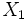$X_1$ and$X_2$are said to be weak homotopy-equivalent or weakly homotopy-equivalent if the following is true:

We can find a collection of topological spaces$X_1 = Y_0, Y_1, Y_2, \dots, Y_n = X_2$ and a collection of continuous maps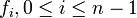$f_i, 0 \le i \le n - 1$, such that each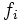$f_i$ is a map either from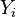$Y_i$ to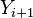$Y_{i+1}$ or a map from$Y_{i+1}$ to$Y_i$, and further, each$f_i$ is a weak homotopy equivalence of topological spaces.

## Relation with other equivalence relations

### Stronger equivalence relations

Equivalence relation Meaning Proof of implication Proof of strictness (reverse implication failure) Intermediate notions
homeomorphic spaces there is a bijective homeomorphism between the spaces
homotopy-equivalent topological spaces there is a homotopy equivalence of topological spaces between the spaces homotopy-equivalent not implies weak homotopy-equivalent

### Weaker equivalence relations

Equivalence relation Meaning Proof of implication Proof of strictness (reverse implication failure) Intermediate notions
Spaces that have isomorphic homotopy groups isomorphic homotopy groups not implies weak homotopy-equivalent
homology-equivalent topological spaces generated by back and forth homology equivalences homology-equivalent not implies weak homotopy-equivalent
Spaces that have isomorphic homology groups and isomorphic fundamental groups and set of path components isomorphic homology groups and isomorphic fundamental groups not implies weak homotopy-equivalent